Next: About this document ... Up: Plotting functions Previous: Plotting functions   Contents

## ellipse.m

Syntax:

[x,y] = ellipse(N10,delN10,N26,delN26,0,plotString)
[h1] = ellipse(N10,delN10,N26,delN26,1,plotString)
[h1,h2] = ellipse(N10,delN10,N26,delN26,2,plotString)


Plots an uncertainty ellipse on the [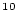Be]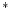- [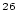Al]/ [Be]diagram (the Lal-Klein-Nishiizumi Al-Be diagram').

The arguments N10, delN10, N26, and delN26 are theBe andAl concentrations and their uncertainties (atoms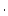g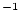).

The third argument can be 0, in which case the function returns the x and y coordinates of the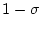uncertainty ellipse; 1, in which case the function plots the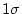uncertainty ellipse and returns its handle; or 2, in which case the function plotsand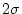uncertainty ellipses and returns their handles. The default is 1.

The argument plotString is an optional string telling the function what line type and color to use to draw the ellipse. See the documentation for the MATLAB function plot` for more information.

What this function actually does is calculate the joint probability on a mesh in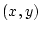: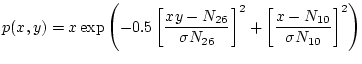(83)

It then creates a normalized cumulative distribution for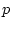to determine the values ofthat account for 68% and 95% of the total probability, and draws contours of the 2-d PDF at those values.Next: About this document ... Up: Plotting functions Previous: Plotting functions   Contents
2007-11-13